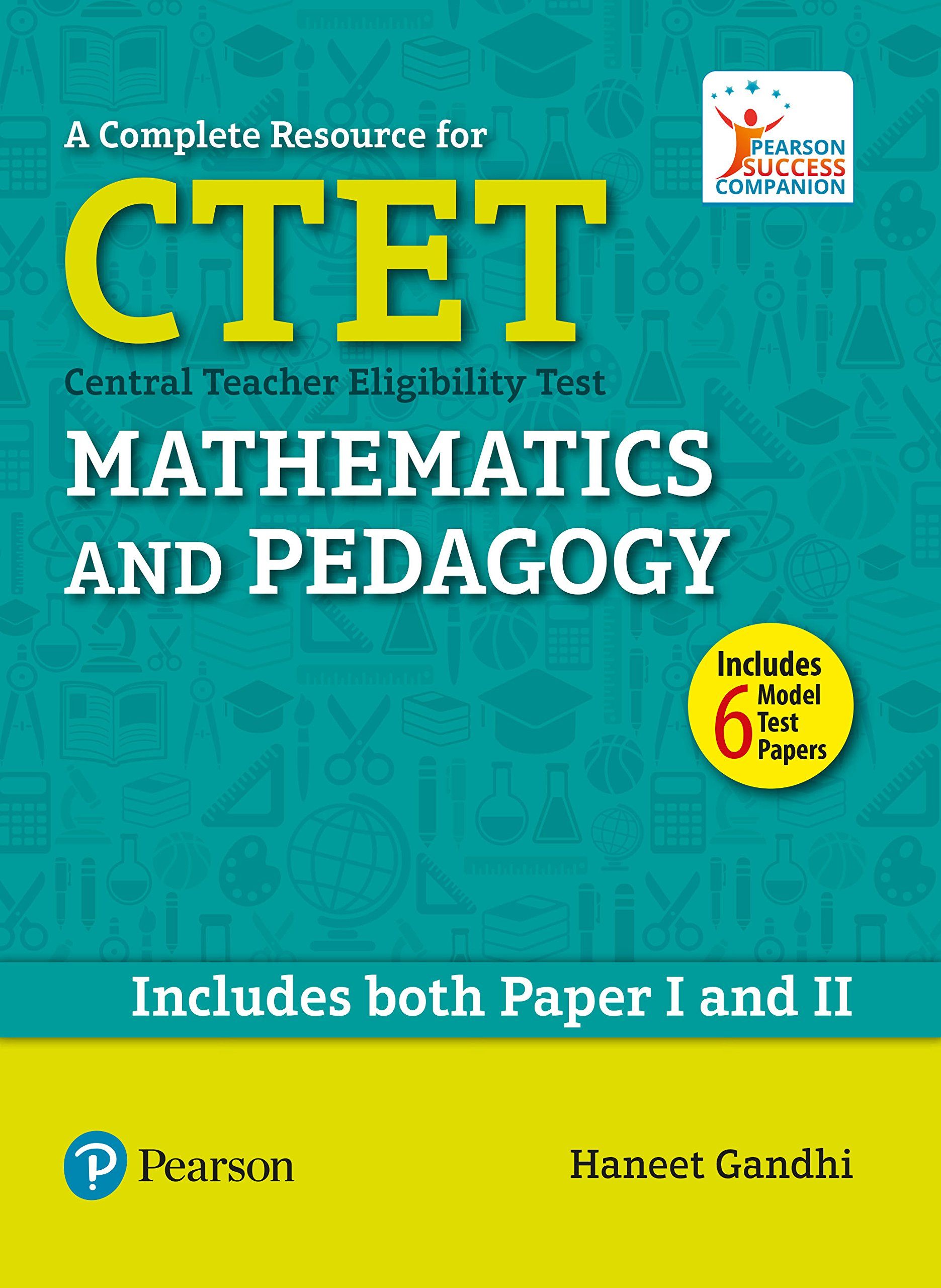# A Complete Resource for CTET: Mathematics and PedagogyPrice: ₹ 350.00
(as of Sep 26,2021 04:34:50 UTC – Details)

The book in your hand, titled Mathematics and its Pedagogy, was conceived to help pre-service and in-service teachers to get acquainted to the salient ideas related to teaching-learning of school mathematics. The book is largely divided in two parts. Part I is for teachers teaching at the primary levels and Part II is for those teaching at the middle grades.

Each part is further divided in two units, making a total of four Units. The first unit of each part covers pedagogy related to the content of mathematics. The topics that cover the syllabus of primary and middle school have been respectively dealt in the first unit of each part. The chapters of Unit I and Unit III intend to introduce the reader to the crucial aspects related to the teaching and learning specific to the content covered at primary and middle school mathematics.

Contents:

1) Numbers
3) Multiplication and Division
4) Length and Angles
5) Shape and Space
6) Area and Volume
7) Weight, Time and Money
8) Introduction to Statistics
9) Patterns

Unit II –
1) How Children Learn Mathematics
2) Concerns in Learning Mathematics
3) Curriculum and Evaluation
4) Common Errors and Their Analysis

Unit III –
2) Fractions
3) Decimals, Percents and Ratios
4) Introduction to Algebra
5) Two-Dimensional Shapes Part-I
6) Two-Dimensional Shapes Part-II
7) Three Dimensional Shapes
8) Data and Chance

Unit IV –
1) Theories of Mathematical Thinking
2) Learning Mathematics
3) Concerns in Learning Mathematics
4) Curriculum and Evaluation
5) Common Errors and Their Analysis